Courses
Courses for Kids
Free study material
Offline Centres
More

# NCERT Solutions for Class 6 Maths Chapter 9 - ExerciseLast updated date: 04th Dec 2023
Total views: 529.8k
Views today: 6.29k

## NCERT Solutions for Class 6 Maths Chapter 9 Data Handling (Ex 9.1) Exercise 9.1

Free PDF download of NCERT Solutions for Class 6 Maths Chapter 9 Exercise 9.1 (Ex 9.1) and all chapter exercises at one place prepared by expert teacher as per NCERT (CBSE) books guidelines. Class 6 Maths Chapter 9 Data Handling Exercise 9.1 Questions with Solutions to help you to revise complete Syllabus and Score More marks. Register and get all exercise solutions in your emails.

## Access NCERT Solutions for Class 6 Mathematics Chapter 9- Data Handling

Exercise 9.1

1. In a mathematics test the following marks were obtained by $40$ students. Arrange these marks in a table using tally marks.

\begin{array}{*{20}{c}}8&1&3&7&6&5&5&4&4&2 \\ 4&9&5&3&7&1&6&5&2&7 \\ 7&3&8&4&2&8&9&5&8&6 \\ 7&4&5&6&9&6&4&4&6&6 \\ \end{array}

Ans: Make a frequency table and denote the frequency of marks by vertical bars.

 Marks Tally Marks No. of Students $1$ || $2$ $2$ ||| $3$ $3$ ||| $3$ $4$ (Image will be uploaded soon) || $7$ $5$ (Images will be uploaded soon) | $6$ $6$ (Image will be uploaded soon)|| $7$ $7$ (Image will be uploaded soon) $5$ $8$ |||| $4$ $9$ ||| $3$

(a) Find how many students obtained marks equal to or more than $7$?

Ans: From the frequency table, the frequency of $7$ marks is five students, frequency of $8$ marks is four students and frequency of $9$ marks is three students. So, $5 + 4 + 3 = 12$ students obtained marks equal to or more than $7$.

(b) How many students obtained marks below $4$?

Ans: : From the frequency table, the frequency of $3$ marks is three students, frequency of $2$ marks is three students and frequency of $1$ mark is two students. So, $2 + 3 + 3 = 8$ students obtained marks below $4$.

2. Following is the choice of sweets of $30$ students of Class VI.

(a) Arrange the names of sweets in a table using tally marks.

Ans: Make a frequency table and denote the frequency of marks by vertical bars.

 Sweets Tally Marks No. of Students Ladoo (Images will be uploaded soon) | $11$ Barfi (Images will be uploaded soon)||| $3$ Jalebi (Image will be uploaded soon) || $7$ Rasgulla (Image will be uploaded soon)|||| $9$ $30$

(b) Which sweet is preferred by most of the students?

Ans: The highest frequency $11$ is from Ladoo, so Ladoo is most preferred.

3. Catherine threw a dice $40$ times and noted the number appearing each time as shown below:

\begin{array}{*{20}{c}} 1&3&5&6&6&3&5&4&1&6 \\ 2&5&3&4&6&1&5&5&6&1 \\ 1&2&2&3&5&2&4&5&5&6 \\ 5&1&6&2&3&5&2&4&1&5&{}&{} \end{array}

Make a table and enter the data using tally marks. Find the number that appeared.

Ans: Make a frequency table and denote the frequency of marks by vertical bars.

 Numbers Tally Marks How many times? $1$ (Image will be uploaded soon) || $7$ $2$ (Image will be uploaded soon) | $6$ $3$ (Image will be uploaded soon) $5$ $4$ |||| $4$ $5$ (Images will be uploaded soon)| $11$ $6$ (Image will be uploaded soon)|| $7$

(a) The minimum number of times.

Ans: The minimum frequency is $4$ of $4$. So, the minimum number of times$= 4$.

(b) The maximum number of times.

Ans: The maximum frequency is $11$ of $5$. So, the maximum number of times$= 5$.

(c) Find those numbers that appear an equal number of times.

Ans: $1$ and $6$ have the same frequency.

4. Following pictograph shows the number of tractors in five villages:

 Villages No. of Tractors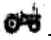- 1 Tractor Village A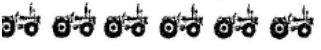Village B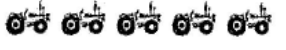Village C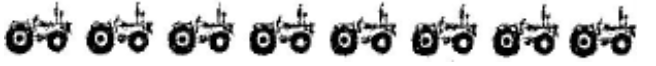Village DVillage E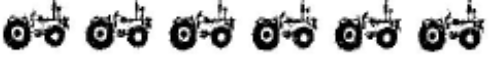Observe the pictograph and answer the following questions:

(i) Which village has the minimum number of tractors?

Ans: Village D has a minimum number of tractors.

(ii) Which village has the maximum number of tractors?

Ans: Village C has a maximum number of tractors.

(iii) How many more tractors village C has as compared to village B.

Ans: Village C has $3$ more tractors then village B.

(iv) What is the total number of tractors in all the five villages?

Ans: The total number of tractors in all villages is $6 + 5 + 8 + 3 + 6 = 28$.

5. The number of girl students in each class of a co-educational middle school is depicted by the pictograph.

 Classes Number of Girl Students- 4 Girls I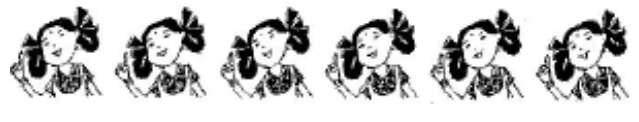II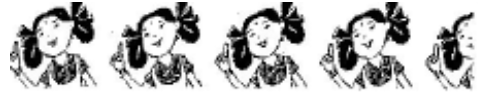III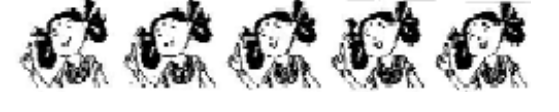IV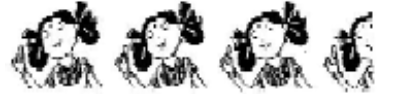V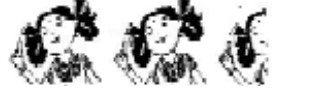VIVII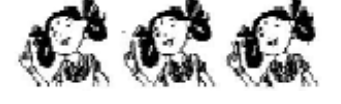VIII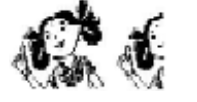Observe this pictograph and answer the following questions:

(a) Which class has the minimum number of girl students?

Ans: The minimum numbers of girl students are in Class VIII.

(b) Is the number of girls in class VI less than the number of girls in class V?

Ans: No, the number of girls in class VI is more than the number of girls in class V since $16 > 10$.

(c) How many girls are there in class VII?

Ans: Each picture represents $4$ girls so the number of girls in class VII is $3 \times 4 = 12$students.

6. The sale of electric bulbs on different days of a week is shown below:

 Days Number of Electric Bulbs- 2 Bulbs Monday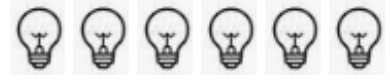Tuesday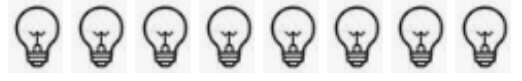Wednesday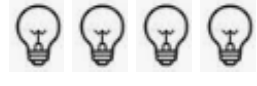ThursdayFriday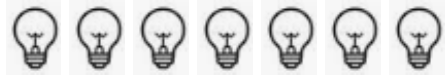Saturday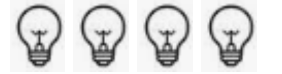Sunday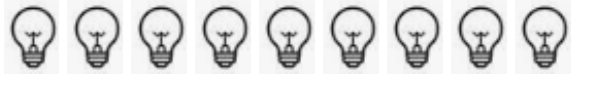Observe the pictograph and answer the following questions:

(a) How many bulbs were sold on Friday?

Ans: Each picture represents $2$ bulbs so sale of bulbs on Friday is $2 \times 7 = 14$ bulbs.

(b) On which day were the maximum numbers of bulbs sold?

Ans: Maximum numbers of bulbs were sold on Sunday.

(c) On which of the days has the same number of bulbs sold?

Ans: On Wednesday and Saturday the same numbers of bulbs were sold.

(d) On which of the days minimum numbers of bulbs were sold?

Ans: On Wednesday and Saturday minimum numbers of bulbs were sold.

(e) If one big carton can hold 9 bulbs. How many cartons were needed in the given week?

Ans: Total number of weeks sold in the whole week is $6 \times 2 + 8 \times 2 + 4 \times 2 + 5 \times 2 + 7 \times 2 + 4 \times 2 + 9 \times 2 = 12 + 16 + 8 + 10 + 14 + 8 + 18 = 86$. Each carton can hold $9$ so the number of cartons required $= 86 \div 9$. Number of cartons required is $10$.

7. In a village six fruit merchants sold the following number of fruit baskets in a particular season:

 Name of Fruit Merchants Number of Fruit Baskets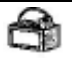- 100 Fruit Baskets Rahim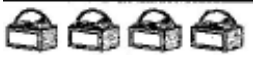Lakhanpal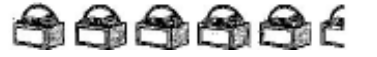AnwarMartin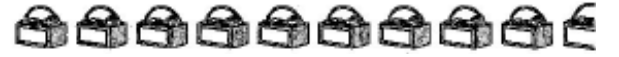Ranjit Singh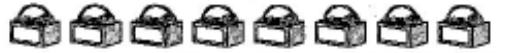Joseph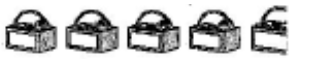Observe this pictograph and answer the following questions:

(a) Which merchant sold the maximum number of baskets?

Ans: Martin sold the highest number of baskets.

(b) How many fruit baskets were sold by Anwar?

Ans: Each picture represents $100$ baskets so the number of baskets sold by Anwar is $100 \times 7 = 700$ students.

(c) The merchants who have sold $600$ or more baskets are planning to buy a godown for the next season. Can you name them?

Ans: Ranjit Singh, Anwar, and Martin are planning to buy a godown for the next season.

## NCERT Solutions for Class 6 Maths Chapter 9 Data Handling Exercise 9.1

Opting for the NCERT solutions for Ex 9.1 Class 6 Maths is considered as the best option for the CBSE students when it comes to exam preparation. This chapter consists of many exercises. Out of which we have provided the Exercise 9.1 Class 6 Maths NCERT solutions on this page in PDF format. You can download this solution as per your convenience or you can study it directly from our website/ app online.

Vedantu in-house subject matter experts have solved the problems/ questions from the exercise with the utmost care and by following all the guidelines by CBSE. Class 6 students who are thorough with all the concepts from the Subject Maths textbook and quite well-versed with all the problems from the exercises given in it, then any student can easily score the highest possible marks in the final exam. With the help of this Class 6 Maths Chapter 9 Exercise 9.1 solutions, students can easily understand the pattern of questions that can be asked in the exam from this chapter and also learn the marks weightage of the chapter. So that they can prepare themselves accordingly for the final exam.

Besides these NCERT solutions for Class 6 Maths Chapter 9 Exercise 9.1, there are plenty of exercises in this chapter which contain innumerable questions as well. All these questions are solved/answered by our in-house subject experts as mentioned earlier. Hence all of these are bound to be of superior quality and anyone can refer to these during the time of exam preparation. In order to score the best possible marks in the class, it is really important to understand all the concepts of the textbooks and solve the problems from the exercises given next to it.

Do not delay any more. Download the NCERT solutions for Class 6 Maths Chapter 9 Exercise 9.1 from Vedantu website now for better exam preparation. If you have the Vedantu app in your phone, you can download the same through the app as well. The best part of these solutions is these can be accessed both online and offline as well.

## FAQs on NCERT Solutions for Class 6 Maths Chapter 9 - Exercise

1. How many questions are present in NCERT Solutions for Class 6 Maths Chapter 9 Data Handling?

The  NCERT Solutions for Class 6 Maths Chapter 9 Data Handling has the following exercise questions-

• Exercise 9.1- seven questions

• Exercise 9.2- two questions

• Exercise 9.3- three questions

• Exercise 9.4- four questions

The solutions to all these exercise questions can be found on the official website of Vedantu. All the solutions are well explained in detail so that students can clear their doubts easily. Students can also access these solutions on the Vedantu app. All the resources are available free of cost.

2. What is data and why is it important to study data handling?

The concept of data is explained in Class 6 Maths Chapter 9 Data Handling. Data is a collection of numbers that provide us with information about something. To understand the information that the data shows we represent it in different forms like tally marks, pictographs or bar diagrams etc. This chapter is important because it clears the fundamentals of what data is and how to represent data in a meaningful way that allows us to interpret the information it provides.

3. How many questions have long solutions (more than 180 words) in NCERT Solutions for Class 6 Maths Chapter 9 Data Handling?

The following exercise questions in NCERT Solutions for Class 6 Maths Chapter 9 Data handling are the long answer type -

• Exercise 9.1- questions 1,2,3 and 6

• Exercise 9.2- questions 1 and 2

• Exercise 9.3- questions 2 and 3

• Exercise 9.4- questions 1,2,3 and 4.

Students can find detailed solutions to these questions on the official website of Vedantu.

4. What are the basics of Class 6 Maths Chapter 9 Data Handling?

Chapter 9 of Class 6 Math teaches the fundamentals of data and how to handle it. Students will learn about the definition of data and how it is represented to make the information meaningful. They will learn the different methods of data representation like tally marks, pictographs, and bar graphs. Students will also learn how to make and read a pictograph and a bar graph. They will learn about choosing a scale while representing data in a bar graph.

5. Which questions and examples are important in class 6 Maths Chapter 9 Data handling?

The following questions should be practised by students to understand and master the chapter Data Handling-

• Arranging numbers using tally marks

• Interpreting pictographs by identifying the maximum and a minimum number of a quantity.

• Drawing a pictograph

• Drawing and interpreting a bar graph

Practising the examples and the exercise questions from this chapter will help students clarify all their doubts and gain confidence in these topics.